﻿ Complex Mathematical Examples In The Notebook As Egyptian Hieroglyphs Stock Photo - Image of math, mathematical: 144011728

# Complex mathematical examples in the notebook as Egyptian hieroglyphs.

## Complex mathematical examples in the notebook as Egyptian hieroglyphs.

More similar stock images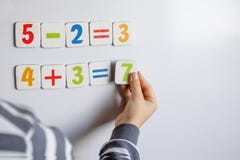The boy solves mathematical examples. A child solves examples on a blackboard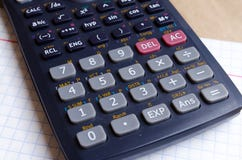Calculator. Maths. School calculations. Two and four. Bugtery Notebook. Office to work in the office. School supplies. Pens, penci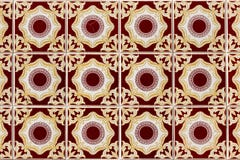Exotic geometric tile patternBeauty in Science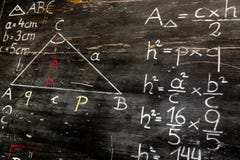Old blackboard with mathematical calculations and drawings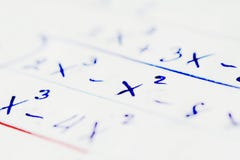Math concept Mathematical thinking using the calculation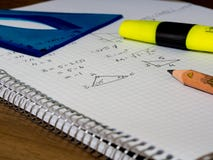Square mathematics student book, and colored pencil on it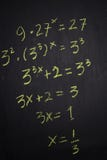Close up math formulas written on a blackboard. Education concept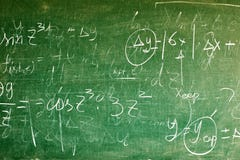Handwritten equations on a green blackboard
More stock photos from Andrii Zastrozhnov's portfolio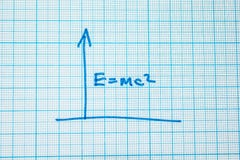The formula is E = mc2 in the notebook per cell. Up arrow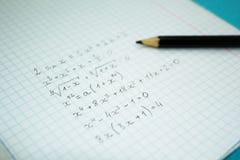Mathematical examples and calculations in a notebook for lectures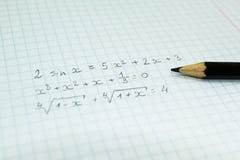Mathematical formulas in a notebook for lectures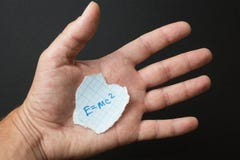The formula E = mc2 in the hand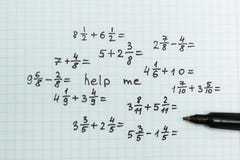The problem in mathematics is help me. Top view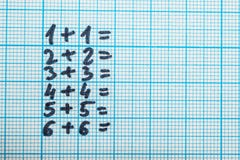Mathematical tasks on paper in a notebook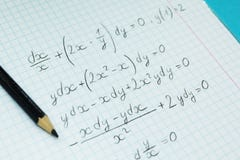Mathematical examples and calculations in a notebook for lectures. The study of arithmetic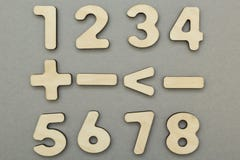Mathematical signs and figures on a gray background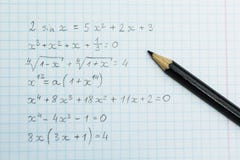Homework on arithmetic. Formulas in the notebook
Related categories